# Worksheet Question & Answers: Integers Notes | Study Mathematics (Maths) Class 6 - Class 6

## Class 6: Worksheet Question & Answers: Integers Notes | Study Mathematics (Maths) Class 6 - Class 6

The document Worksheet Question & Answers: Integers Notes | Study Mathematics (Maths) Class 6 - Class 6 is a part of the Class 6 Course Mathematics (Maths) Class 6.
All you need of Class 6 at this link: Class 6

Q.1. Write 'True' or 'False' for the following :
(i) –20° C represent temperature "above 20°C"
(ii) 8 > (–10)
(iii) | – 2 | = 2
(iv) | – 4 – 2 | = – 6 (v) (–3) > (–5)

Ans.
(i) False
(ii) True
(iii) True
(iv) False
(v) True

Q.2. Fill up the blanks :
(i) Integer which is neither positive nor negative is ____________
(ii) Predecessor of (–99) is ____________
(iii) | -26-14 | = ____________
(iv) Successor of (–100) is ____________
(v) Largest 3-digit negative integer is ____________

Ans.
(i) 0
(ii) –100
(iii) 40
(iv) –99
(v) –100

Q.3. Subtract (–25) from the sum of 15 and 35.
Ans.
75

Q.4. Add the sum of (–2063) and 562 to (–2063).
Ans.
–3564

Q.5. Simplify :
(i) (–50) + (–200) – (–500)
(ii) 23 – (–15) + 12
(iii) (24 + 6) ÷ (–3)
(iv) 19 + {10 ÷ (7 – 9)}
(v) 12 – {16 – (6 + 2 – 6 ÷ 3)}

Ans.
(i) 250
(ii) 50
(iii) (–10)
(iv) 14
(v) 2

Q.6. Write additive inverse of :
(i) (–6347)
(ii) 0
(iii) 4231
(iv) 2132 – 132
(v) –10 – 5

Ans.
(i) 6347
(ii) 0
(iii) –4231
(iv) –2000
(v) 15

Q.7.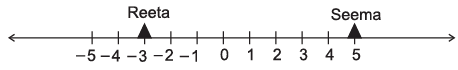Observe the number line given above and answer the following :
(i) What is the position of Reeta on the number line?
(ii) What is the position of Seema?
(iii) What is the distance between Reeta and Seema?

Ans.
(i) Position of Reeta = –3
(ii) Position of Seema = 5
(iii) Distance between them = 8 units

Q.8. Represent the following numbers/expressions on a number line?
(i) –7
(ii) + 9
(iii) 7 – 2
(iv) – 5–4
(v) 4 – 9

Ans.

(i)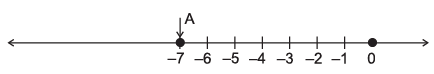(ii)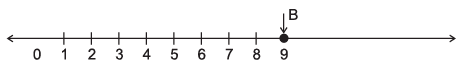(iii)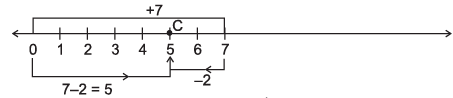(iv)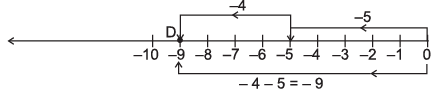(v)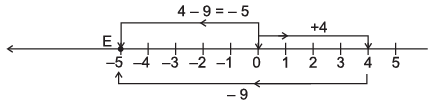Q.9. Enter the correct sysmbol >, =, < in the following :
(i) (–7) _________  (2)
(ii) 7 _________  (–12)
(iii) 0 _________  (–2)
(iv) (–16) _________  (9 + 7)
(v) – (10 + 5) _________  (–15)

Ans.
(i) <
(ii) >
(iii) >
(iv) <
(v) =

Q.10. Find the values of 'a' when :
(i) a + 10 = – 18
(ii) a – 3 = 7
(iii) –13a = 91
(iv) a ÷ 5 = 3

Ans.
(i) –28
(ii) 10
(iii) –7
(iv) 15

The document Worksheet Question & Answers: Integers Notes | Study Mathematics (Maths) Class 6 - Class 6 is a part of the Class 6 Course Mathematics (Maths) Class 6.
All you need of Class 6 at this link: Class 6Use Code STAYHOME200 and get INR 200 additional OFF

## Mathematics (Maths) Class 6

239 videos|259 docs|43 tests

Track your progress, build streaks, highlight & save important lessons and more!

,

,

,

,

,

,

,

,

,

,

,

,

,

,

,

,

,

,

,

,

,

;